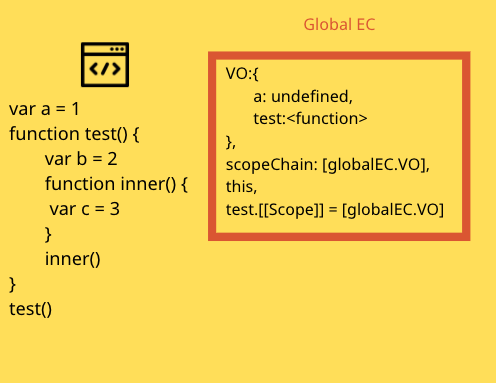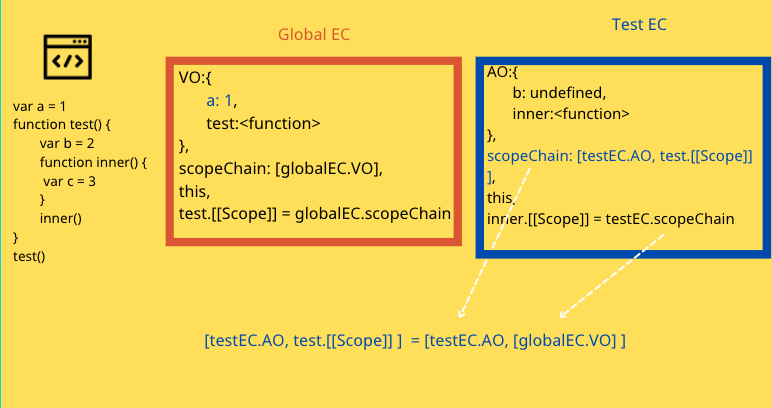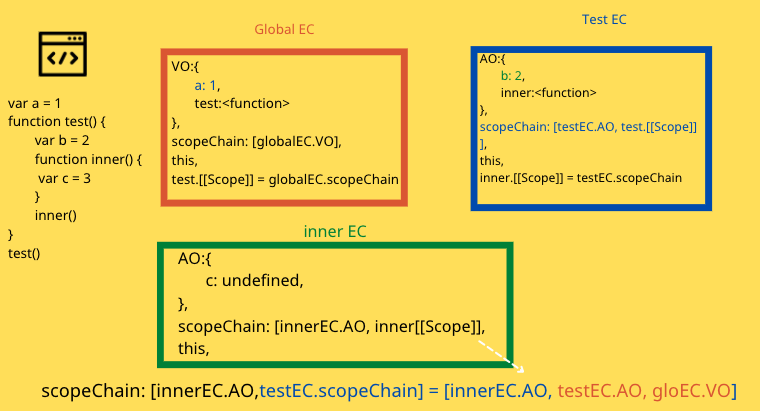# JavaScript 程式執行原理：Scope Chain 與 var, let, const

## EC 裡面裝些什麼？Scope Chain 怎麼來的 ？

EC: {
VO{
a:undefined
}
}

function test() {
var a = 666
}

test()


EC 初始化後，進入執行階段後會變這樣：

EC: {
VO{
a:666
}
}

function test() {
var a = 666
}

test()


EC: {
VO{
a:666,
b:undefined

}
}

function test(a, b) {
//...
}

test(666)


EC: {
VO{
a:666,
b:undefined,
c:function

}
}

function test(a, b) {
//...
function c () { //... }
c()
}

test(666)


EC: {
VO{
a: <function>,

}
}

function test(a) {

function a () { //... }

}

test(666)


EC: {
VO{
a: 666,
b: <function>,
c: 888
}
}

function test(a,b) {
function b () { //... }
var a = 777 // 被跳過了
var c = 888

}

test(666)


function EC 跟 global EC 一樣 裡面放著 variable object 、function EC 的 Scope Chain (自己的AO + function EC的 [[Scope]] )、this、以及如果有遇到 function 的宣告就產生的 [[ Scope ]]

var a = 1
function test() {
var b = 2
function inner() {
var c = 3
}
inner()
}
test()testEC.scopeChaine = [testEC.AO, test.[[Scope]] ] = [testEC.AO, [globalEC.VO] ]

inner.[[Scope]] = testEC.scopeChainscopeChain: [innerEC.AO,testEC.scopeChain] = [innerEC.AO, testEC.AO, gloEC.VO]

## hoisting

console.log(b) // undefined
var b = 666


var b
console.log(b)
b = 666


，也會順利執行 test function 。

test()
function test(){
//....
}


var a = 666
function test(){
console.log(a)
var a = 777
}
test()


function test(){
console.log(a) // <function>
var a = 777
function a() {
console.log('OMG')
}
}
test()


function test(a){
console.log(a) // 666
var a = 777
console.log(a) // 777
}

test(666)


function test(a){
console.log(a) // <function>
function a(){
}
}

test(666)


function fn(){
fn2()
function fn2(){
b='OMG'
}
}
fn()
console.log(b) // OMG


### let, const 與 var

let, const 與 var 最大的不同是， var 變數的生存範圍是以 function 區塊來界定，然而 const, let 是以 block 來界定的。

let a = 666
function test(){
console.log(a)
let a = 777
}
test()


let 與 const 其實是有 hoisting 的，但不是像 var 一樣，初始化時會是 undefined，並且在賦值以前如果去存取，會拋出錯誤。

let a = 666
function test(){
// ======= a TDZ 開始
console.log(a)
.
.
.
let a = 777 // ======= a TDZ 結束
}
test()

let a = 666
function test(){
test2() // ======= a TDZ 開始
let a = 777 // ======= a TDZ 結束
funciton test2(){
console.log(a)
}
}
test()